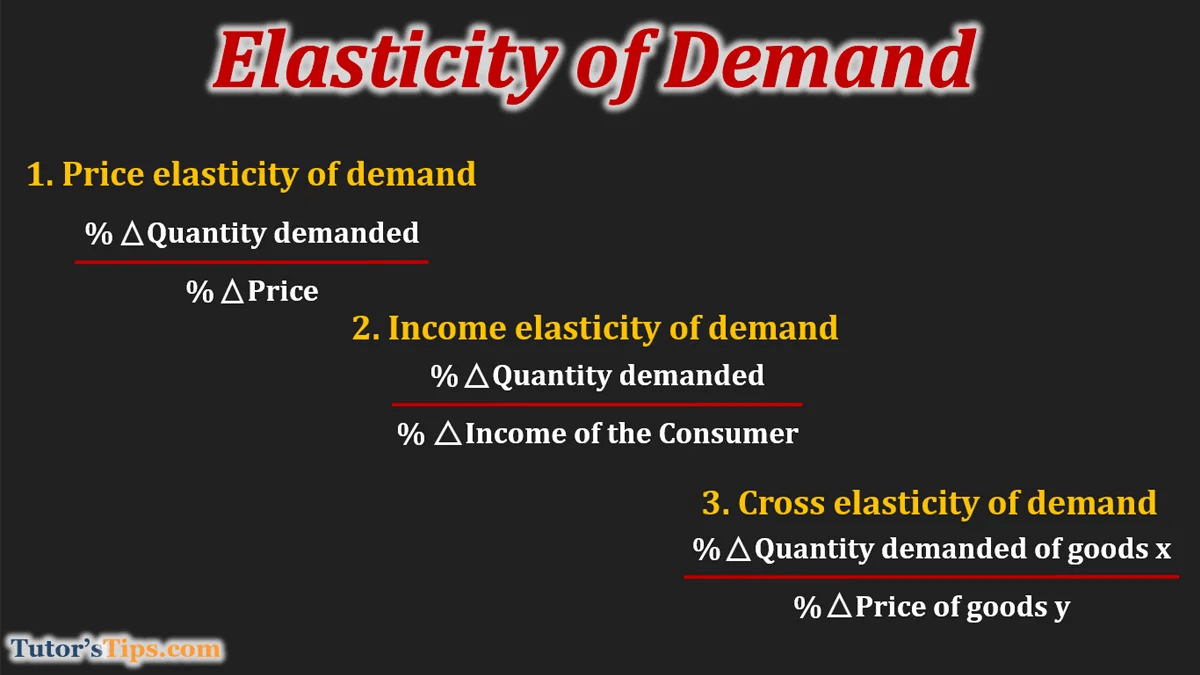# Elasticity of demand – Meaning and its typesThe Elasticity of demand is an economic term that refers to the sensitivity of demand of a good to changes in other economic variables such as the price of goods, price of related goods and income of consumers, etc. In other words, it is the responsiveness of quantity demanded of a product to changes in one of the variables on which demand depends.

It can be calculated as the percentage change in quantity demanded divided by percentage change in another economic variable.% Δ quantity demanded = percentage change in quantity demanded% Δ Economic Variable = percentage change in Economic Variable

A higher value for elasticity of demand with respect to any economic variable means that customers are more responsive to changes in that variable.

For example, prices of rice fall from rs.120/kg to Rs 100/kg. Due to this,  the quantity demanded in the market increases from 100kg to 120 kgs. It implies the response of demand to decline in the price of rice.

### Types of elasticity of demand :

1. Price elasticity of demand
2. Income elasticity
3. Cross elasticity

#### 1. Price elasticity of demand:

It refers to the degree of responsiveness of quantity demanded with respect to change in the price of that particular commodity, other things remain constant.

it can be calculated as the percentage change in quantity demanded divided by the percentage change in price.% Δ quantity demanded = percentage change in quantity demanded

% Δ Price  = percentage change in price

#### 2. Income elasticity of demand:

It refers to the ratio of the percentage of change in quantity demanded and percentage change in income level of consumer. It measures the degree of sensitivity of quantity demanded to change in income.

Hence,

It can be calculated as the percentage change in quantity demanded divided by the percentage change in income of the consumer.% Δ quantity demanded = percentage change in quantity demanded% Δ Income of Consumer = percentage change in Income of Consumer

Higher the income elasticity, more sensitive will be the demand with respect to income.

#### 3. Cross elasticity of demand:

It refers to the sensitivity of demand for one product to the price of another related product. It is the ratio of the percentage change in quantity demanded of goods x and the percentage change in the price of goods y.% Δ quantity demanded of goods x = percentage change in quantity demanded% Δ Price of goods y  = percentage change in Income of Consumer